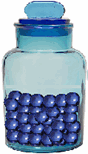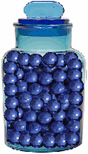Skill 3D
Estimating: Benchmark Numbers

A benchmark is simply using one thing to estimate another.  Benchmarks can be anything - such as pencils, paper clips, shoes - etc. When using benchmarks for measurement, the student does need to know how to look at something and then come up with a proportion.  For example, is the amount half?  Is it double?  Is it triple the amount?  etc. So, in the problem below, we ask the student to look at the first jar and estimate how many will be in the 2nd jar based on the number in the first. This skill is actually not too difficult for most students. Since 100 gumballs are in the first jar, we would then logically estimate that there are 200 gumballs in the 2nd jar as it is twice the size as the first.

If 100 gumballs are in the first jar,
about many gumballs would be in the second jar?